## MTSO May News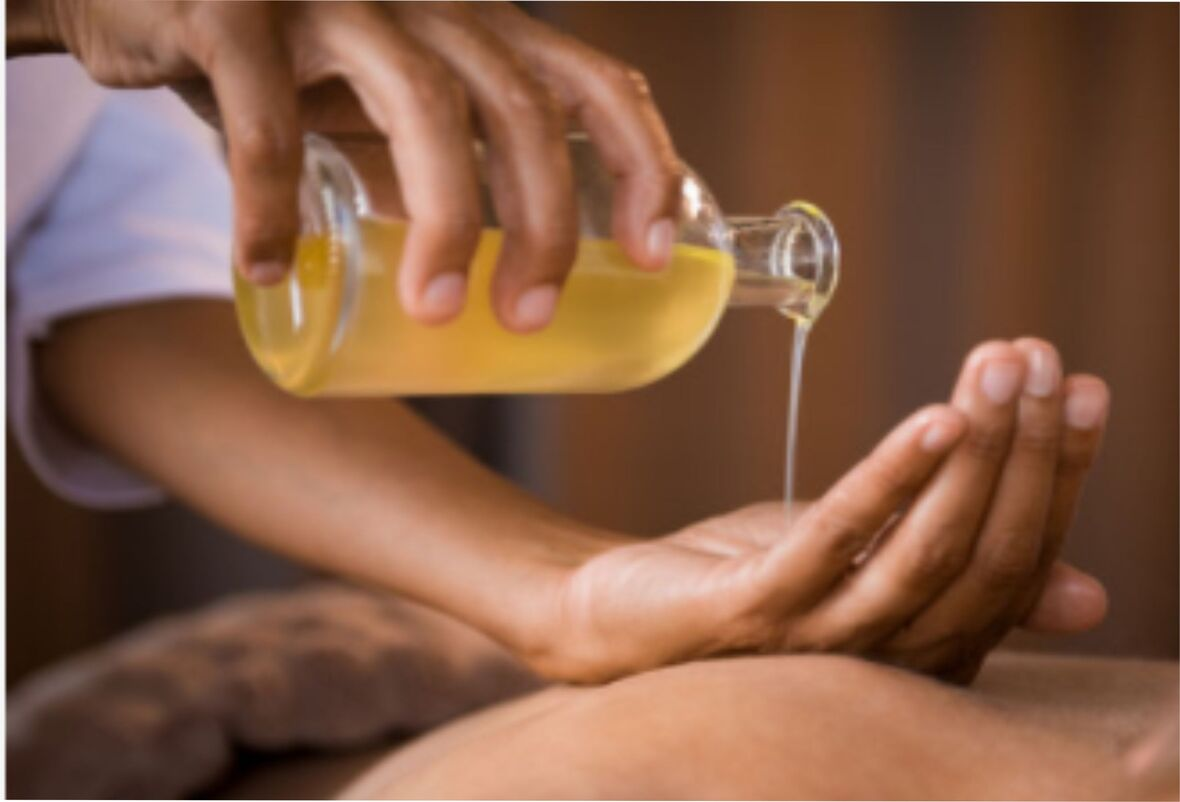Summer is on it's way, blossoms and leaves are starting appear! New beginnings and fresh starts as we move forward to a new season. This is the perfect time to see what needs to be upgraded in your practice, MTSO is here to assist you with our 25 years of experience in the profession. Feel confident knowing you're using only the best quality products with your clients.

Looking for upgrades to your clinic or home office? Call us at 780-440-1818 for suggestions on how to update your workspace with an affordable electric table, a sweet aromatherapy program, or new sheets.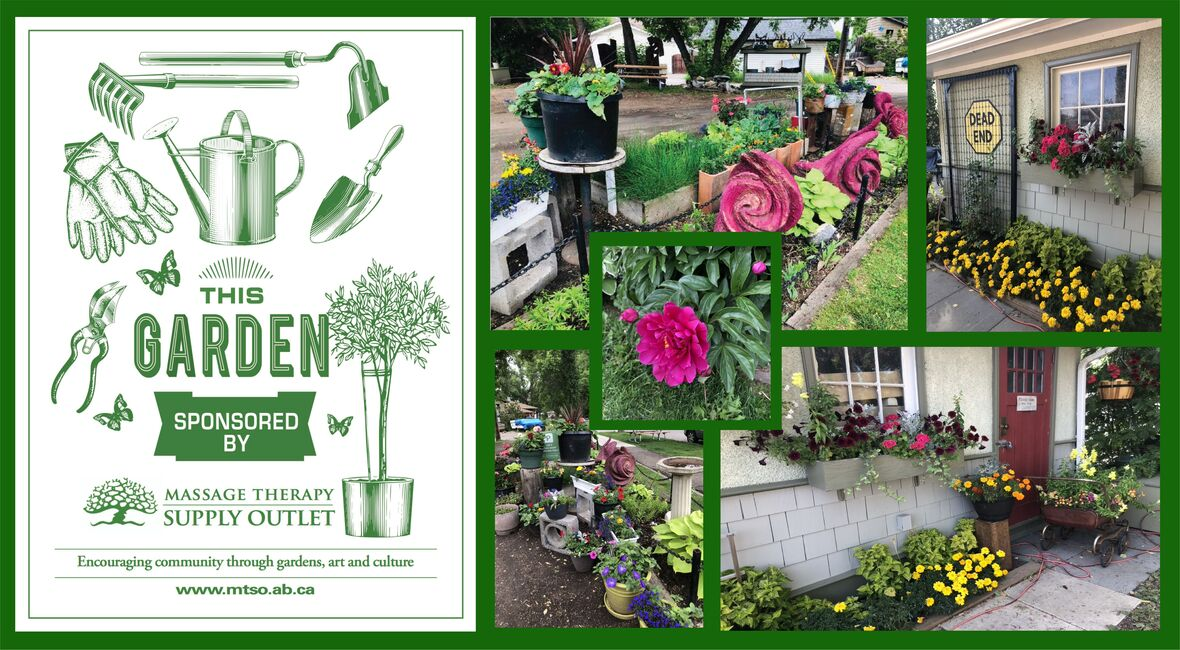# MTSO Garden

The MTSO Family has been busy in our garden, here are a few images from last year's garden. MTSO is about encouraging community through gardens, art and culture

 table div table+table+table+table+table+table div table{width:100%;padding:0}table div table+table+table+table+table+table div table img{width:96.23%;padding:0;float:none}table div table+table+table+table+table+table div table td{width:100%;padding:0 1.88% 18px}/* styles */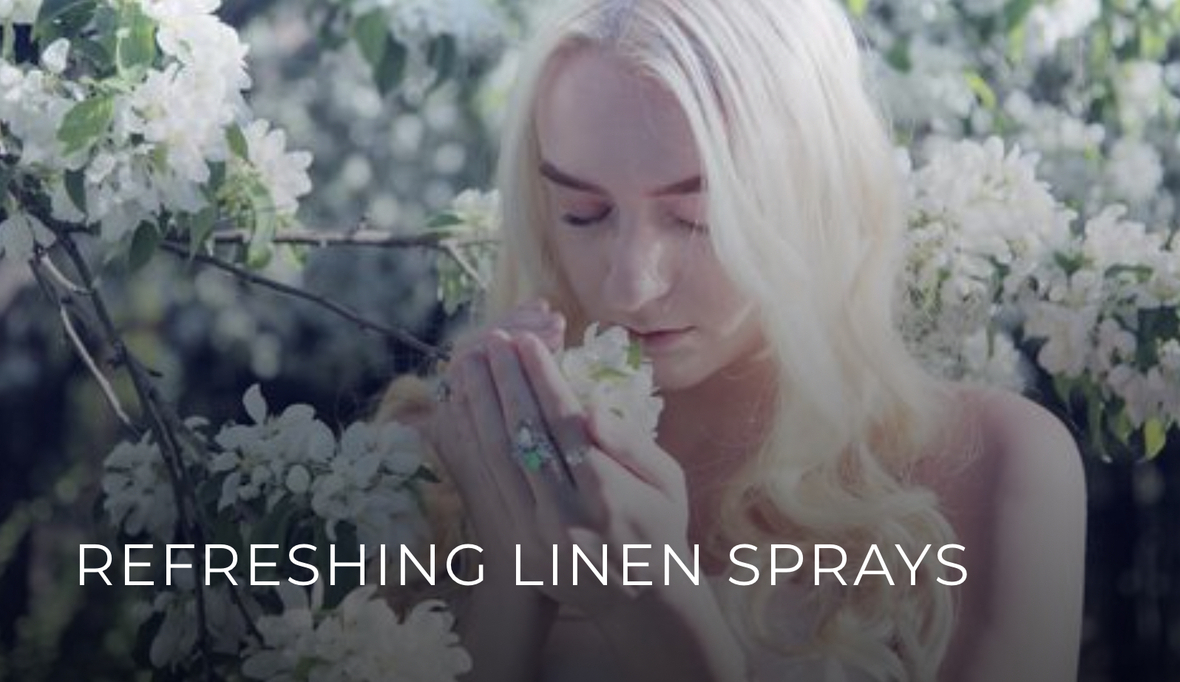# These sprays will work as "air fresheners" or "linen sprays", and when sprayed on linens act to freshen the whole room.

 /* styles */ Into a 120ml bottle with a spray top, add 2-3 tbsp of 99% alcohol USP, and ONE of the following essential oil recipes: 1: Lemongrass 10 drops, Sweet Orange or Grapefruit 20 drops 2: Lavender 15 drops, Lemon 15 drops 3: Lemongrass 20 drops, either Japanese Mint, or Peppermint or (Spearmint): 10 drops 4: Our pre-blended Clarity Synergy (30 drops in the alcohol) is also a terrific & easy way to make a linen/room spray. Once you've measured your essential oils into the alcohol, shake vigorously. Then fill the bottle about 3/4 full with water & shake well again. Shake well before each use. Spray 2 or 3 sprays onto your massage table on top of the linens, once the table is set for the next client. Use sparingly to avoid too much scent or wetting your set up. Enjoy!
 /* styles */
 table div table+table+table+table+table+table+table+table+table+table+table+table div table{width:100%;padding:0}table div table+table+table+table+table+table+table+table+table+table+table+table div table img{width:96.23%;padding:0;float:none}table div table+table+table+table+table+table+table+table+table+table+table+table div table td{width:100%;padding:0 1.88% 18px}/* styles */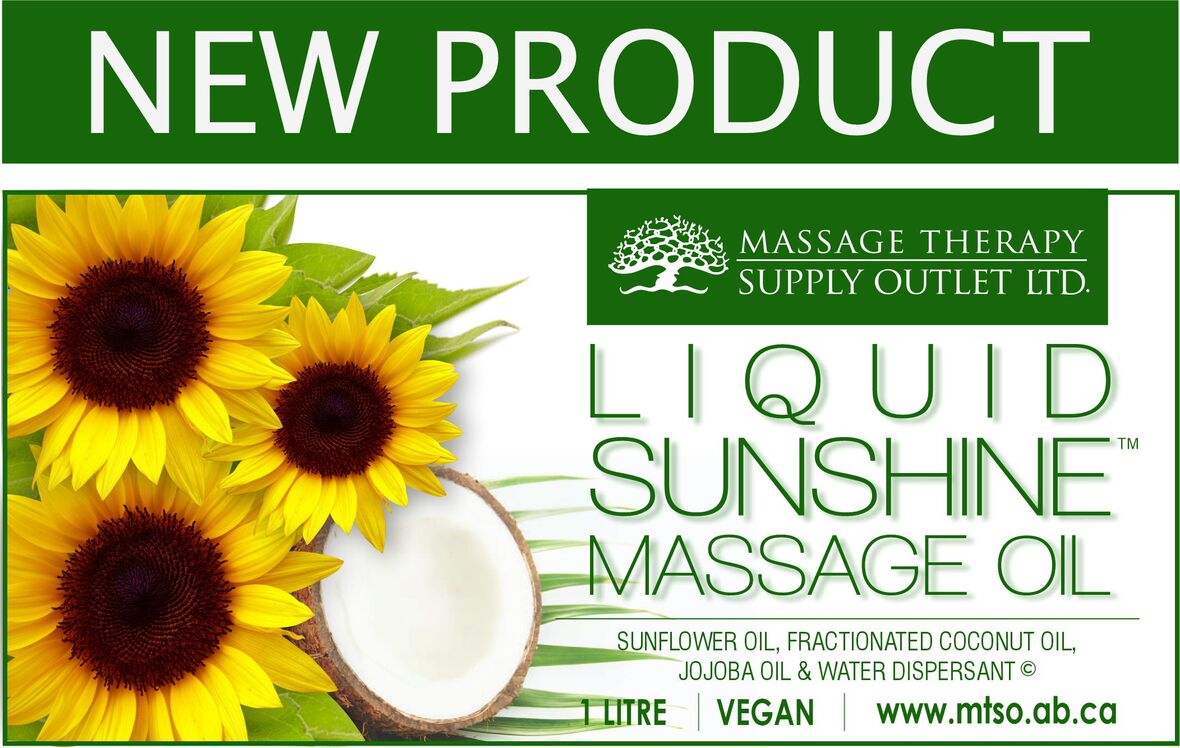# LIQUID SUNSHINE™ MASSAGE OIL 1 LITRE

An ideal natural blend of 3 oils providing nourishing skin care for northern climates. This nut-free, unscented oil is suitable for a wide variety of massage applications.

▪ Sunflower Seed oil, high in vitamins A, B, D & E, minerals & unsaturated fatty acids, is light and easily absorbed.
▪ Fractionated Coconut oil, also high in vitamin E, is a smooth, emollient, easily absorbed oil, providing consistent glide and excellent transport for Aromatherapy oils.
▪ Jojoba oil being rich in vitamins A, D & E, is also soothing and emollient.
▪ The effect is that both client and therapist finish with smooth moisturized skin.
▪ The inclusion of a natural water dispersant assures easy laundering.
 ▪ Sunflower Seed oil, high in vitamins A, B, D & E, minerals & unsaturated fatty acids, is light and easily absorbed.
 ▪ Fractionated Coconut oil, also high in vitamin E, is a smooth, emollient, easily absorbed oil, providing consistent glide and excellent transport for Aromatherapy oils.
 ▪ Jojoba oil being rich in vitamins A, D & E, is also soothing and emollient.
 ▪ The effect is that both client and therapist finish with smooth moisturized skin.
 ▪ The inclusion of a natural water dispersant assures easy laundering.

Available in 2 sizes: 1 litre & 4 litres

 table div table+table+table+table+table+table+table+table+table+table+table+table+table+table+table+table div table{width:100%;padding:0}table div table+table+table+table+table+table+table+table+table+table+table+table+table+table+table+table div table img{width:96.23%;padding:0;float:none}table div table+table+table+table+table+table+table+table+table+table+table+table+table+table+table+table div table td{width:100%;padding:0 1.88% 18px}/* styles */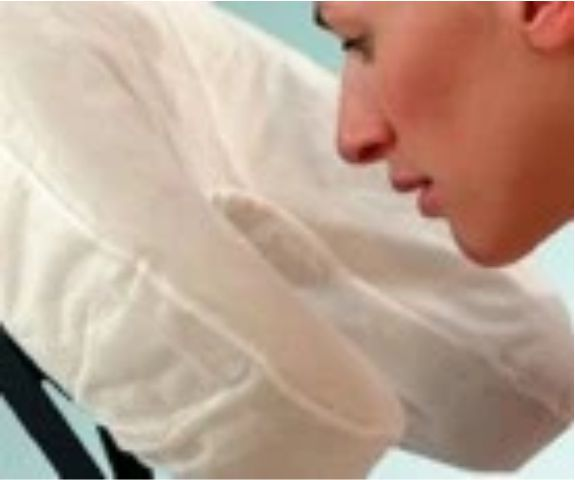Fitted Disposable Face Cradle Covers

# FITTED DISPOSABLE FACE CRADLE COVERS

Made from soft, 25 gsm spunbound fabric, this new and innovative design provides a comfortable, sanitary and disposable barrier for your clients and an easy changeover for between client protection.

▪ Fits all crescent-shaped face rests
▪ Stays put throughout your treatment
▪ Great for table and seated massage
▪ Box of 50
▪ Latex Free

 table div table+table+table+table+table+table+table+table+table+table+table+table+table+table+table+table+table+table+table div table{width:100%;padding:0}table div table+table+table+table+table+table+table+table+table+table+table+table+table+table+table+table+table+table+table div table img{width:96.23%;padding:0;float:none}table div table+table+table+table+table+table+table+table+table+table+table+table+table+table+table+table+table+table+table div table td{width:100%;padding:0 1.88% 18px}/* styles */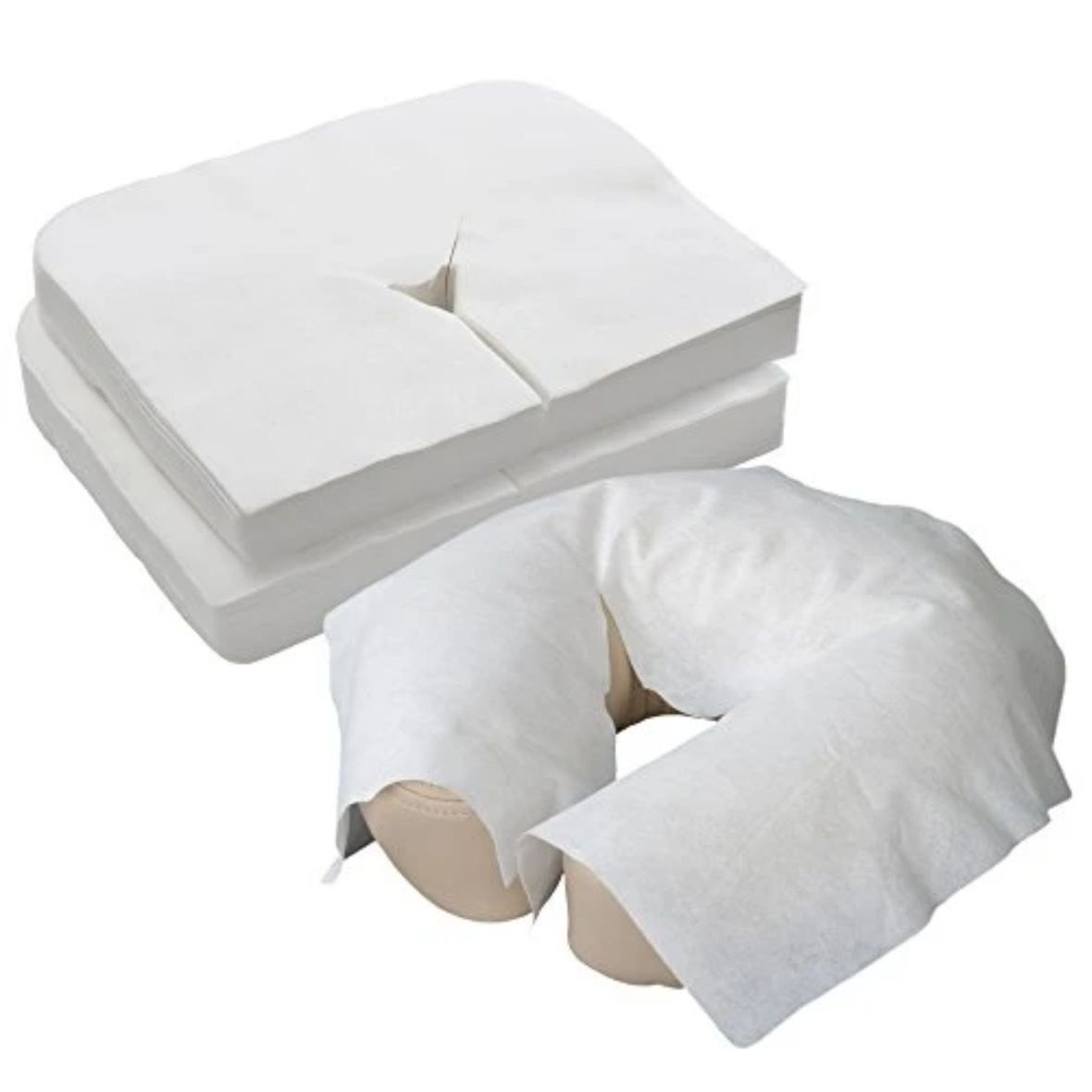Flat Disposable Face Cradle Covers

# FLAT DISPOSABLE FACE CRADLE COVERS

Flat soft disposable face cradle covers package of 100. Product stores easily in table and chair carry cases. Thick, yet soft and silky fabric conforms to the shape of your cushion. Feels like napped cotton. Flat face covers fit almost anything.

 table div table+table+table+table+table+table+table+table+table+table+table+table+table+table+table+table+table+table+table+table+table+table div table{width:100%;padding:0}table div table+table+table+table+table+table+table+table+table+table+table+table+table+table+table+table+table+table+table+table+table+table div table img{width:96.23%;padding:0;float:none}table div table+table+table+table+table+table+table+table+table+table+table+table+table+table+table+table+table+table+table+table+table+table div table td{width:100%;padding:0 1.88% 18px}/* styles */# SHEET SET - FLANNELETTE

\$36.95

▪ Made in 100% cotton flannelette
▪ The Flat Sheets are 42" - 43" wide and 88" long.
▪ The Fitted Sheets are made to fit tables up to 32" wide.
▪ The full set Includes fitted sheet, flat sheet and face cradle cover.
 ▪ Made in 100% cotton flannelette
 ▪ The Flat Sheets are 42" - 43" wide and 88" long.
 ▪ The Fitted Sheets are made to fit tables up to 32" wide.
 ▪ The full set Includes fitted sheet, flat sheet and face cradle cover.

 table div table+table+table+table+table+table+table+table+table+table+table+table+table+table+table+table+table+table+table+table+table+table+table+table+table div table{width:100%;padding:0}table div table+table+table+table+table+table+table+table+table+table+table+table+table+table+table+table+table+table+table+table+table+table+table+table+table div table img{width:96.23%;padding:0;float:none}table div table+table+table+table+table+table+table+table+table+table+table+table+table+table+table+table+table+table+table+table+table+table+table+table+table div table td{width:100%;padding:0 1.88% 18px}/* styles */table.module-25{width:95.85%;padding:0}table div table+table+table+table+table+table+table+table+table+table+table+table+table+table+table+table+table+table+table+table+table+table+table+table+table+table div table{width:95.85%;float:none;margin-left:auto;margin-right:auto;padding:0}table div table+table+table+table+table+table+table+table+table+table+table+table+table+table+table+table+table+table+table+table+table+table+table+table+table+table div table a{border:0 none;text-decoration:none}table div table+table+table+table+table+table+table+table+table+table+table+table+table+table+table+table+table+table+table+table+table+table+table+table+table+table div table img{width:100%!important;border:0 none;text-decoration:none}table div table+table+table+table+table+table+table+table+table+table+table+table+table+table+table+table+table+table+table+table+table+table+table+table+table+table div table td{width:100%;padding:0}/* styles */
 table div table+table+table+table+table+table+table+table+table+table+table+table+table+table+table+table+table+table+table+table+table+table+table+table+table+table+table+table div table{width:100%;padding:0}table div table+table+table+table+table+table+table+table+table+table+table+table+table+table+table+table+table+table+table+table+table+table+table+table+table+table+table+table div table img{width:96.23%;padding:0;float:none}table div table+table+table+table+table+table+table+table+table+table+table+table+table+table+table+table+table+table+table+table+table+table+table+table+table+table+table+table div table td{width:100%;padding:0 1.88% 18px}/* styles */# STORE CLOSED for Victoria Day

We will be closed on Monday, May 24 for Victoria Day. We'll be open regular hours 10am to 6pm on Tuesday, May 25. Our online store is 24/7 www.mtso.ab.ca

 table div table+table+table+table+table+table+table+table+table+table+table+table+table+table+table+table+table+table+table+table+table+table+table+table+table+table+table+table+table+table div table{width:100%;padding:0}table div table+table+table+table+table+table+table+table+table+table+table+table+table+table+table+table+table+table+table+table+table+table+table+table+table+table+table+table+table+table div table img{width:96.23%;padding:0;float:none}table div table+table+table+table+table+table+table+table+table+table+table+table+table+table+table+table+table+table+table+table+table+table+table+table+table+table+table+table+table+table div table td{width:100%;padding:0 1.88% 18px}/* styles */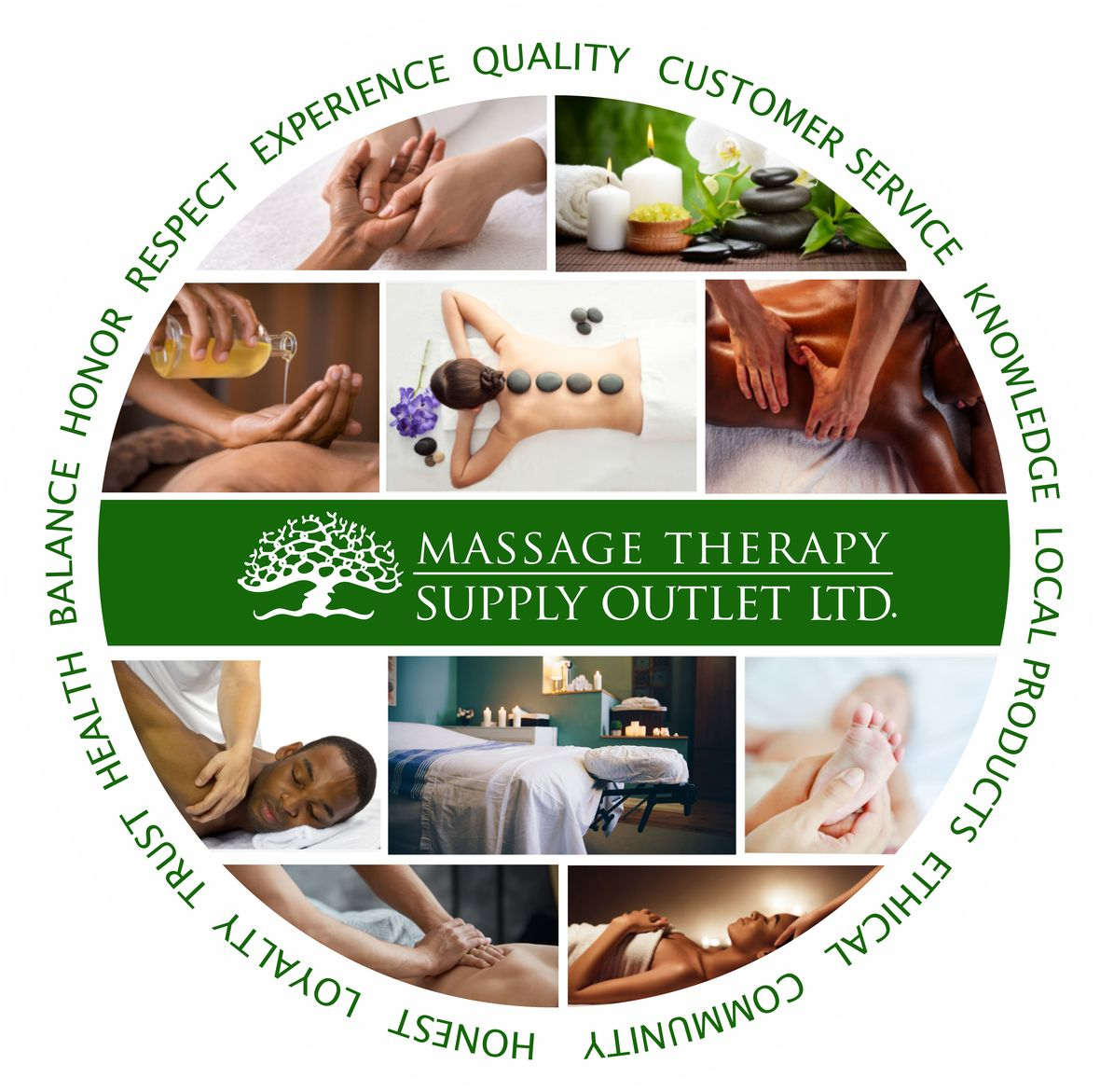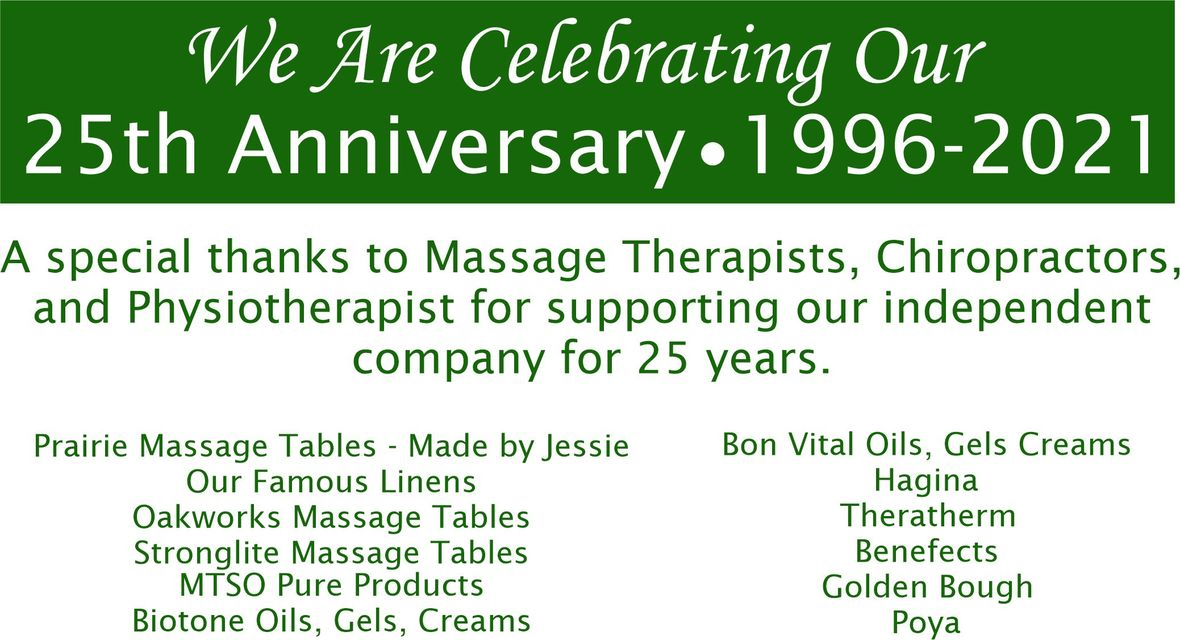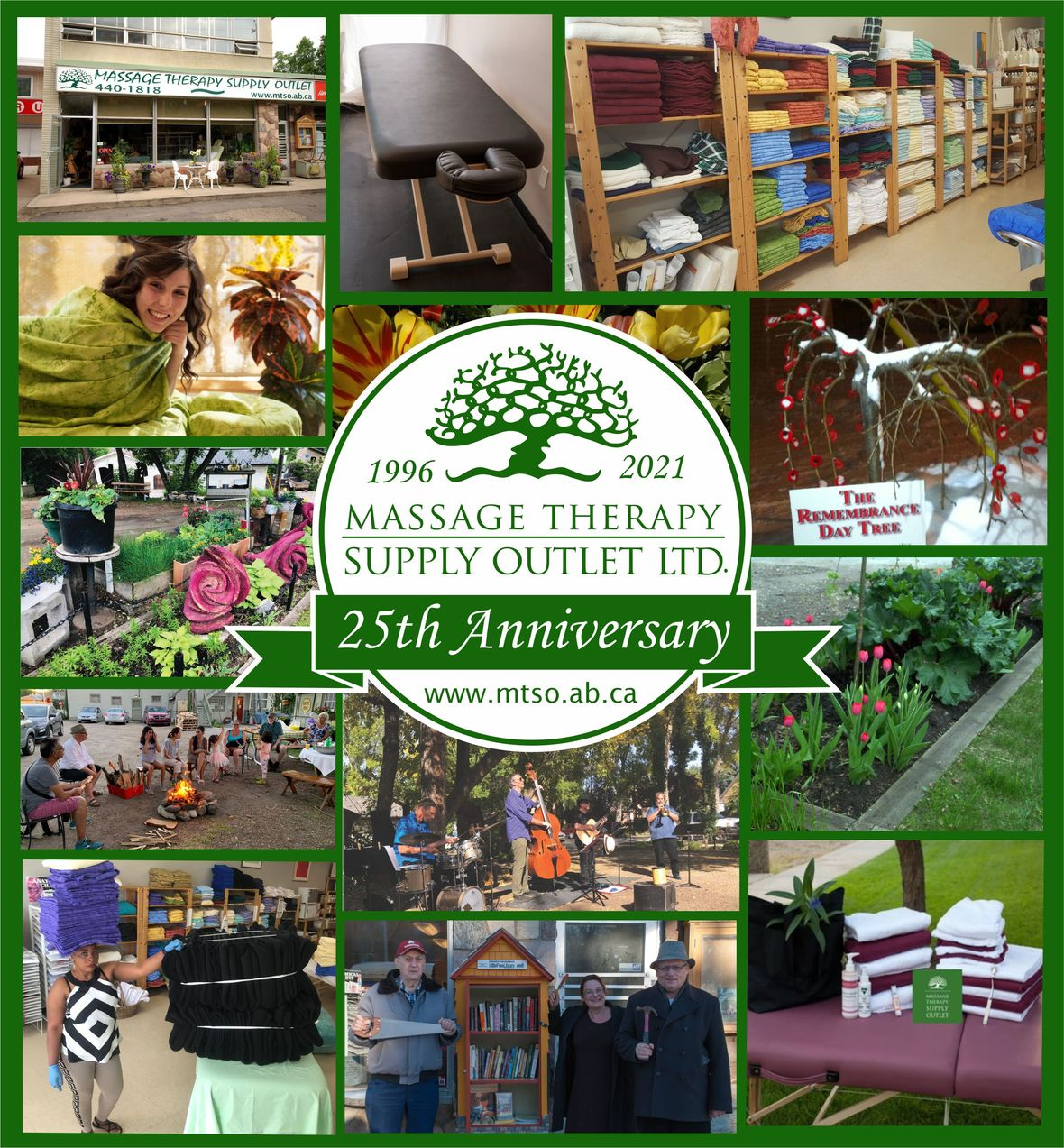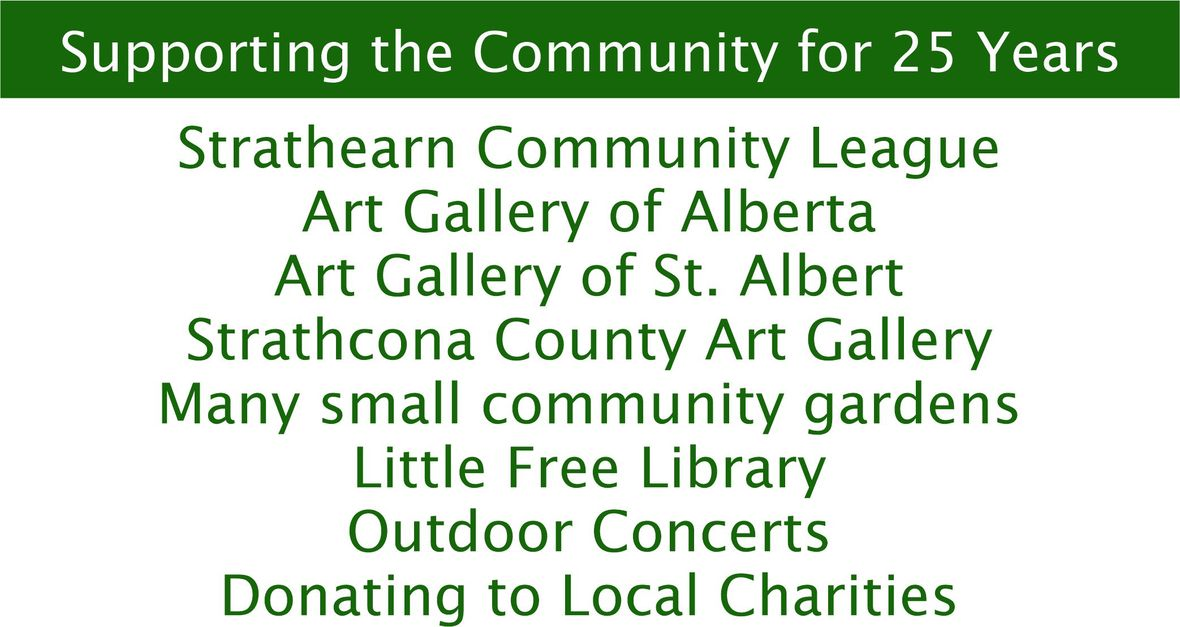table div table+table+table+table+table+table+table+table+table+table+table+table+table+table+table+table+table+table+table+table+table+table+table+table+table+table+table+table+table+table+table+table+table+table+table div table{width:100%;padding:0}table div table+table+table+table+table+table+table+table+table+table+table+table+table+table+table+table+table+table+table+table+table+table+table+table+table+table+table+table+table+table+table+table+table+table+table div table img{width:96.23%;padding:0;float:none}table div table+table+table+table+table+table+table+table+table+table+table+table+table+table+table+table+table+table+table+table+table+table+table+table+table+table+table+table+table+table+table+table+table+table+table div table td{width:100%;padding:0 1.88% 18px}/* styles */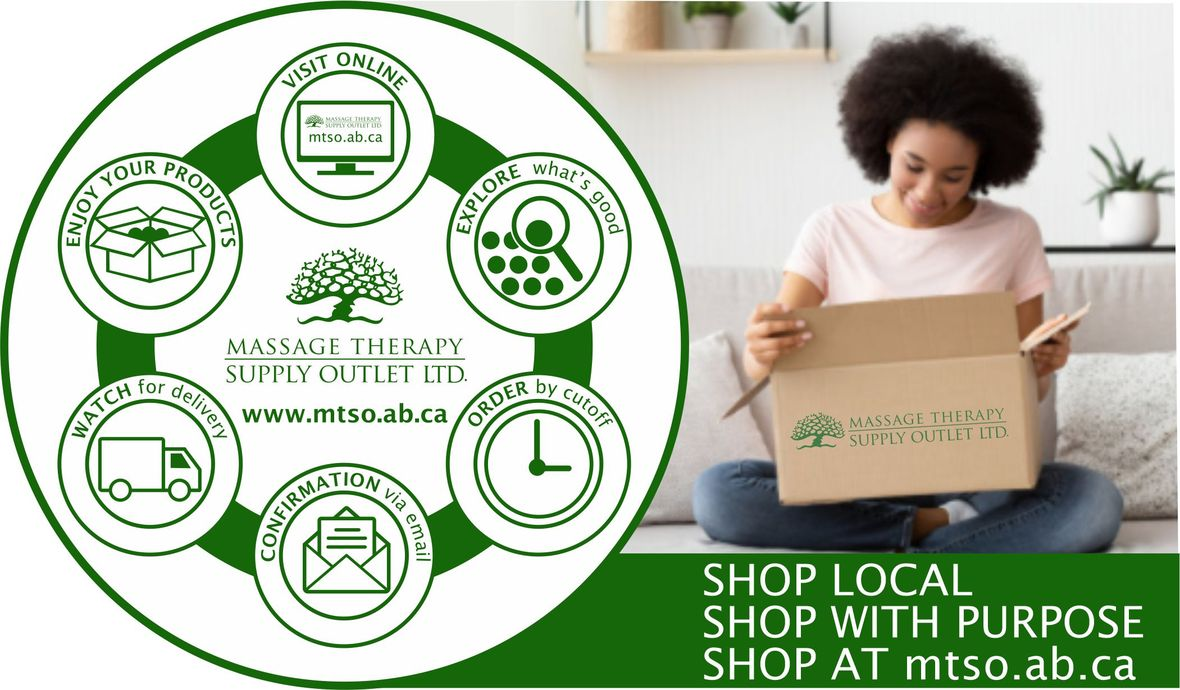# CURBSIDE PICKUP IS AVAILABLE

In addition to in-store shopping and shipping, curbside pick-up is also available. You can either phone-in your order or place order online. When placing your order online choose Local Pick Up at check out. We will contact you when your order is ready.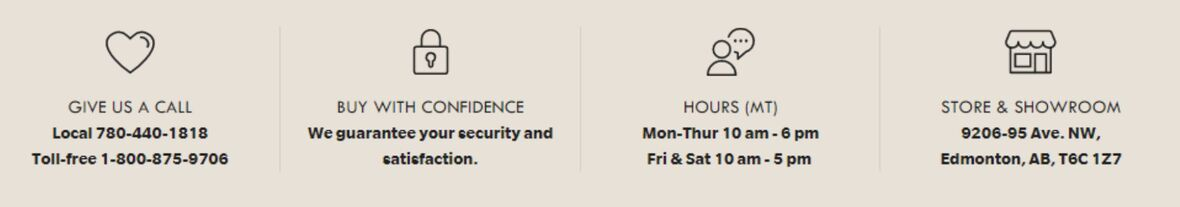/* styles */ Browse and buy from the extensive collection of quality products at our online store, always open, 24/7/365. Drop by our Edmonton store and showroom: 10:00 - 6:00 Mon-Thur, 10:00-5:00 Fri & Sat.
 Like   Tweet   Pin   in